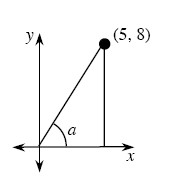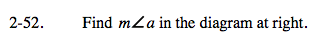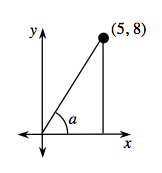### Home > AC > Chapter 14 > Lesson 14.2.1.4 > Problem2-52

2-52.

Find ma in the diagram below. Homework Help ✎The coordinate of the point gives the leg lengths of the triangle.

Use tangent to find the angle.

$\text{tan}(a)=\frac{8}{5}$Solve.

ma = 57.99°